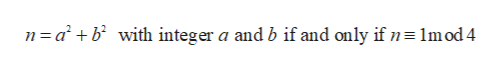# If a, b,n are integers and a^2 + b^2 = n, then the remainder of n upon division by 4 is not 3.

Question
9 views

If a, b,n are integers and a^2 + b^2 = n, then the remainder of n upon division by 4 is not 3.

check_circle

Step 1

Here a and b are two integer then n is the sum of two square. There is only one case n to be prime is a = b = 1 otherwise there is no prime.

If n is any number and if it is divided by 4 then remainder is 3 so, we can represent the number n as:

Step 2

Using the statement of the Format’s theorem on the sum of two squares stat...help_outlineImage Transcriptionclosen abwith integer a and b if and only if n 1m od 4 fullscreen

### Want to see the full answer?

See Solution

#### Want to see this answer and more?

Solutions are written by subject experts who are available 24/7. Questions are typically answered within 1 hour.*

See Solution
*Response times may vary by subject and question.
Tagged in

### Math